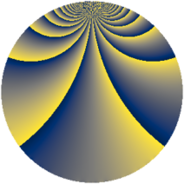# Properties

 Label 1849.4.kLevel $1849$ Weight $4$ Character orbit 1849.k Rep. character $\chi_{1849}(6,\cdot)$ Character field $\Q(\zeta_{129})$ Dimension $39648$ Sturm bound $630$

# Related objects

## Defining parameters

 Level: $$N$$ $$=$$ $$1849 = 43^{2}$$ Weight: $$k$$ $$=$$ $$4$$ Character orbit: $$[\chi]$$ $$=$$ 1849.k (of order $$129$$ and degree $$84$$) Character conductor: $$\operatorname{cond}(\chi)$$ $$=$$ $$1849$$ Character field: $$\Q(\zeta_{129})$$ Sturm bound: $$630$$

## Dimensions

The following table gives the dimensions of various subspaces of $$M_{4}(1849, [\chi])$$.

Total New Old
Modular forms 39816 39816 0
Cusp forms 39648 39648 0
Eisenstein series 168 168 0

## Trace form

 $$39648q - 84q^{2} - 81q^{3} - 3860q^{4} - 67q^{5} - 101q^{6} - 35q^{7} - 14q^{8} + 4189q^{9} + O(q^{10})$$ $$39648q - 84q^{2} - 81q^{3} - 3860q^{4} - 67q^{5} - 101q^{6} - 35q^{7} - 14q^{8} + 4189q^{9} - 113q^{10} - 120q^{11} - 14q^{12} - 75q^{13} - 3192q^{14} - 151q^{15} - 15004q^{16} + 4q^{17} - 333q^{18} - 3389q^{19} + 409q^{20} - 68q^{21} - 466q^{22} + 3527q^{23} - 288q^{24} + 11615q^{25} - 65q^{26} + 108q^{27} + 708q^{28} - 33q^{29} + 5006q^{30} - 2869q^{31} + 712q^{32} + 338q^{33} + 145q^{34} + 9868q^{35} + 17638q^{36} + 43q^{37} + 1112q^{38} - 1468q^{39} - 1431q^{40} - 1028q^{41} - 148q^{42} - 1111q^{43} - 520q^{44} - 1974q^{45} - 46q^{46} + 830q^{47} + 2315q^{48} + 22667q^{49} - 510q^{50} - 1676q^{51} + 414q^{52} + 203q^{53} + 1034q^{54} + 1156q^{55} + 179q^{56} + 1840q^{57} - 1414q^{58} + 2840q^{59} + 499q^{60} - 523q^{61} - 1595q^{62} + 2136q^{63} - 57746q^{64} + 2040q^{65} - 2538q^{66} + 714q^{67} + 8361q^{68} + 3417q^{69} + 84q^{70} + 1459q^{71} + 77952q^{72} - 1378q^{73} + 2244q^{74} + 89964q^{75} + 2918q^{76} - 1534q^{77} - 5816q^{78} + 1579q^{79} + 3071q^{80} + 36362q^{81} - 48490q^{82} - 423q^{83} - 8426q^{84} - 2032q^{85} - 2862q^{86} + 26448q^{87} + 5286q^{88} + 2110q^{89} + 103498q^{90} + 3427q^{91} - 2299q^{92} + 897q^{93} - 33184q^{94} + 4848q^{95} - 2604q^{96} - 58992q^{97} + 127q^{98} + 17695q^{99} + O(q^{100})$$

## Decomposition of $$S_{4}^{\mathrm{new}}(1849, [\chi])$$ into newform subspaces

The newforms in this space have not yet been added to the LMFDB.